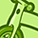# QlikView App Dev

Discussion Board for collaboration related to QlikView App Development.

Announcements
Now Live: Qlik Sense SaaS Simplified Authoring – Analytics Creation for Everyone: READ DETAILS
cancel
Showing results for
Did you mean:Not applicable

## Current Month and Previous Month

Hi All,

I need to take current and previous month sales based on the selected month and year

My Sales Month  looks like this 1,2,3,4,5,6,7,8,9,10,11,12

My Sales Yerar looks like this 2011,2012,2013

To calculate current month sales i used this expression below (based on selected month),

=

sum({<SALES_MONTH={"\$(=max(SALES_MONTH))"}>} \$(vSales)

It works but when i try to calculate the previous month using the expression below, value is coming as "0"

=

sum({<SALES_MONTH={"\$(=max(SALES_MONTH)-1)"}>} \$(vSales)

Kindly guide where i'm wrong in this expression

Thanks,

Selva

1 Solution

Accepted SolutionsMVP

Create a numeric month field in the script: num(month(date#(MonthName,'MMM'))) as MonthNumber

You'll also need an incremental month field because the monthnum of Jan is 1 and the previous month is not 0, but 12 in the previous year. You can add a field like this: Year*12+num(month(date#(MonthName,'MMM'))) as PeriodNumber. You can then use the PeriodNumber field in the set analysis expressions:

Current: sum({<Sales_Year=,Sales_Month=,PeriodNumber={\$(=max(PeriodNumber))>} Sales)

Previous Month: sum({<Sales_Year=,Sales_Month=,PeriodNumber={\$(=max(PeriodNumber)-1)>} Sales)

Same Month previous year: sum({<Sales_Year=,Sales_Month=,PeriodNumber={\$(=max(PeriodNumber)-12)>} Sales)

talk is cheap, supply exceeds demand
7 RepliesNot applicable
Author

for current--

sum({<SalesMonth={"\$(=(SalesMonth))"},SalesYear={"\$(=(SalesYear))"}>}Sales)

for previous--

sum({<SalesMonth={"\$(=(SalesMonth)-1)"},SalesYear={"\$(=(SalesYear))"}>}Sales)Creator III

PFA. Hope it helps.

Regards,

SomNot applicable
Author

Hi Som,

Suppose my Sales month look like this ( Jan,Feb,Mar,Apr etc to Dec)

How to calculate the previous year.

Thanks,

SelvaMaster

Hi Selva,

Your expresion look fine. Can you tell me the definition of \$(vSales)?

Regards,

SokkornMVP

Create a numeric month field in the script: num(month(date#(MonthName,'MMM'))) as MonthNumber

You'll also need an incremental month field because the monthnum of Jan is 1 and the previous month is not 0, but 12 in the previous year. You can add a field like this: Year*12+num(month(date#(MonthName,'MMM'))) as PeriodNumber. You can then use the PeriodNumber field in the set analysis expressions:

Current: sum({<Sales_Year=,Sales_Month=,PeriodNumber={\$(=max(PeriodNumber))>} Sales)

Previous Month: sum({<Sales_Year=,Sales_Month=,PeriodNumber={\$(=max(PeriodNumber)-1)>} Sales)

Same Month previous year: sum({<Sales_Year=,Sales_Month=,PeriodNumber={\$(=max(PeriodNumber)-12)>} Sales)

talk is cheap, supply exceeds demandNot applicable
Author

Thanks GysbertCreator III

Dear Selva,

In that case, still you can make a DATE using your table fields.

Like Date(Date#('2012-Jan-01','YYYY-MMM-DD'),'DD/MM/YYYY')

Regards,

Som